In an isolated parallel plate capacitor of capacitance C, the four surface have charges Q1, Q2, Q3 and Q4 as shown. The potential difference between the plates is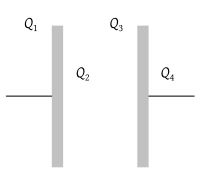(1) $\frac{{Q}_{1}+{Q}_{2}+{Q}_{3}+{Q}_{4}}{2C}$

(2) $\frac{{Q}_{2}+{Q}_{3}}{2C}$

(3) $\frac{{Q}_{2}-{Q}_{3}}{2C}$

(4) $\frac{{Q}_{1}+{Q}_{4}}{2C}$

Concept Questions :-

Combination of capactiors
High Yielding Test Series + Question Bank - NEET 2020

Difficulty Level:

For the circuit shown, which of the following statements is true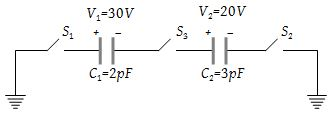(1) With S1 closed, V1 = 15 V, V2 = 20 V

(2) With S3 closed V1 = V2 = 25 V

(3) With S1 and S2 closed V1 = V2 = 0

(4) With S1 and S3 closed, V1 = 30 V, V2 = 20 V

Concept Questions :-

Combination of capactiors
High Yielding Test Series + Question Bank - NEET 2020

Difficulty Level:

Consider the situation shown in the figure. The capacitor A has a charge q on it whereas B is uncharged. The charge appearing on the capacitor B a long time after the switch is closed is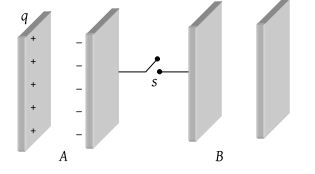(1) Zero

(2) q/2

(3) q

(4) 2q

Concept Questions :-

Combination of capactiors
High Yielding Test Series + Question Bank - NEET 2020

Difficulty Level:

A capacitor of capacitance C1 = 1 μF can withstand maximum voltage V1 = 6kV (kilo-volt) and another capacitor of capacitance C2 = 3 μF can withstand maximum voltage V2 = 4 kV. When the two capacitors are connected in series, the combined system can withstand a maximum voltage of

(1) 4 kV

(2) 6 kV

(3) 8 kV

(4) 10 kV

Concept Questions :-

Combination of capactiors
High Yielding Test Series + Question Bank - NEET 2020

Difficulty Level:

In the given figure each plate of capacitance C has partial value of charge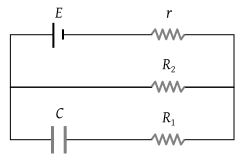(1) CE

(2) $\frac{CE{R}_{1}}{{R}_{2}-r}$

(3) $\frac{CE{R}_{2}}{{R}_{2}+r}$

(4) $\frac{CE{R}_{1}}{{R}_{1}-r}$

Concept Questions :-

RC circuit
High Yielding Test Series + Question Bank - NEET 2020

Difficulty Level:

The plates of a capacitor are charged to a potential difference of 320 volts and are then connected across a resistor. The potential difference across the capacitor decays exponentially with time. After 1 second the potential difference between the plates of the capacitor is 240 volts, then after 2 and 3 seconds the potential difference between the plates will be

(1) 200 and 180 V

(2) 180 and 135 V

(3) 160 and 80 V

(4) 140 and 20 V

Concept Questions :-

RC circuit
High Yielding Test Series + Question Bank - NEET 2020

Difficulty Level:

The plates of a parallel plate condenser are pulled apart with a velocity v. If at any instant their mutual distance of separation is d, then the magnitude of the time of rate of change of capacity depends on d as follows

(1) 1/d

(2) 1/d2

(3) d2

(4) d

Concept Questions :-

Types of capactiors
High Yielding Test Series + Question Bank - NEET 2020

Difficulty Level:

A fully charged capacitor has a capacitance ‘C’. It is discharged through a small coil of resistance wire embedded in a thermally insulated block of specific heat capacity ‘s’ and mass ‘m’. If the temperature of the block is raised by ‘ΔT’, the potential difference ‘V’ across the capacitance is

(1) $\frac{ms\Delta T}{C}$

(2) $\sqrt{\frac{2ms\Delta T}{C}}$

(3) $\sqrt{\frac{2mC\Delta T}{s}}$

(4) $\frac{mC\Delta T}{s}$

Concept Questions :-

Energy stored in capacitor
High Yielding Test Series + Question Bank - NEET 2020

Difficulty Level:

A network of four capacitors of capacity equal to ${C}_{1}=C,\text{\hspace{0.17em}\hspace{0.17em}}{C}_{2}=2C,\text{\hspace{0.17em}}{C}_{3}=3C$ and C4 = 4 C are connected in a battery as shown in the figure. The ratio of the charges on C2 and C4 is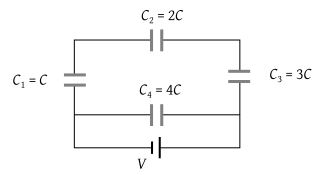(1) $\frac{22}{3}$

(2) $\frac{3}{22}$

(3) $\frac{7}{4}$

(4) $\frac{4}{7}$

Concept Questions :-

Combination of capactiors
High Yielding Test Series + Question Bank - NEET 2020

Difficulty Level:

A 4 μF capacitor, a resistance of 2.5 MΩ is in series with 12 V battery. Find the time after which the potential difference across the capacitor is 3 times the potential difference across the resistor. [Given ln(2)= 0.693]

(1) 13.86 s

(2) 6.93 s

(3) 7 s

(4) 14 s

Concept Questions :-

RC circuit